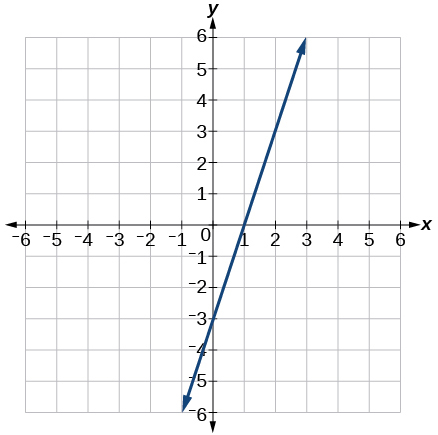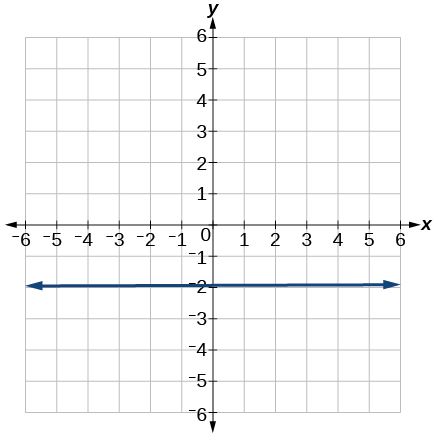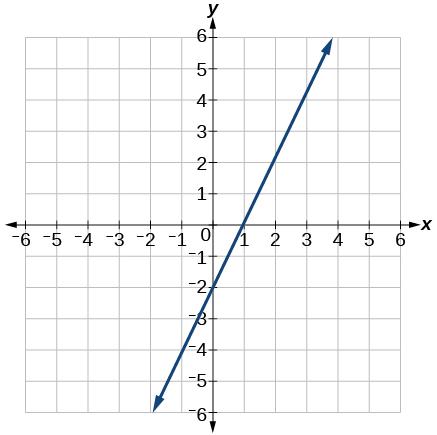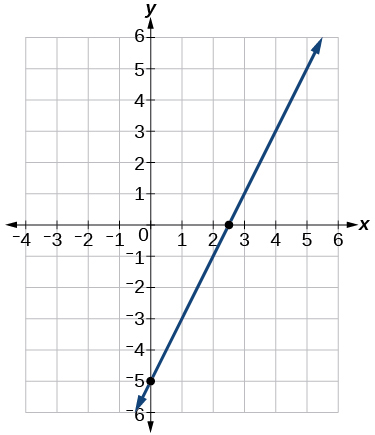# 4.3 Fitting linear models to data  (Page 7/14)

 Page 7 / 14

Determine whether the algebraic equation is linear. $\text{\hspace{0.17em}}6{x}^{2}-y=5$

Determine whether the function is increasing or decreasing.

$f\left(x\right)=7x-2$

Increasing

Determine whether the function is increasing or decreasing.

$g\left(x\right)=-x+2$

Given each set of information, find a linear equation that satisfies the given conditions, if possible.

Passes through $\text{\hspace{0.17em}}\left(\text{7},\text{5}\right)\text{\hspace{0.17em}}$ and $\text{\hspace{0.17em}}\left(\text{3},\text{17}\right)$

$y=-\text{3}x+\text{26}$

Given each set of information, find a linear equation that satisfies the given conditions, if possible.

x -intercept at $\text{\hspace{0.17em}}\left(\text{6},0\right)\text{\hspace{0.17em}}$ and y -intercept at $\text{\hspace{0.17em}}\left(0,\text{1}0\right)$

Find the slope of the line shown in the graph.3

Find the slope of the line graphed.Write an equation in slope-intercept form for the line shown.$y=\text{2}x-\text{2}$

Does the following table represent a linear function? If so, find the linear equation that models the data.

 x –4 0 2 10 g(x) 18 –2 –12 –52

Does the following table represent a linear function? If so, find the linear equation that models the data.

 x 6 8 12 26 g(x) –8 –12 –18 –46

Not linear.

On June 1 st , a company has $4,000,000 profit. If the company then loses 150,000 dollars per day thereafter in the month of June, what is the company’s profit n th day after June 1 st ? For the following exercises, determine whether the lines given by the equations below are parallel, perpendicular, or neither parallel nor perpendicular: $\begin{array}{c}2x-6y=12\\ -x+3y=1\end{array}$ parallel $\begin{array}{c}y=\frac{1}{3}x-2\\ 3x+y=-9\end{array}$ For the following exercises, find the x - and y - intercepts of the given equation $7x+9y=-63$ $\left(–9,0\right);\left(0,–7\right)$ $f\left(x\right)=2x-1$ For the following exercises, use the descriptions of the pairs of lines to find the slopes of Line 1 and Line 2. Is each pair of lines parallel, perpendicular, or neither? Line 1: Passes through $\text{\hspace{0.17em}}\left(5,11\right)\text{\hspace{0.17em}}$ and $\text{\hspace{0.17em}}\left(10,1\right)$ Line 2: Passes through $\text{\hspace{0.17em}}\left(-1,3\right)\text{\hspace{0.17em}}$ and $\text{\hspace{0.17em}}\left(-5,11\right)$ Line 1: $\text{\hspace{0.17em}}m=-2;$ Line 2: $\text{\hspace{0.17em}}m=-2;$ Parallel Line 1: Passes through $\text{\hspace{0.17em}}\left(8,-10\right)\text{\hspace{0.17em}}$ and $\text{\hspace{0.17em}}\left(0,-26\right)$ Line 2: Passes through $\text{\hspace{0.17em}}\left(2,5\right)\text{\hspace{0.17em}}$ and $\text{\hspace{0.17em}}\left(4,4\right)$ Write an equation for a line perpendicular to $\text{\hspace{0.17em}}f\left(x\right)=5x-1\text{\hspace{0.17em}}$ and passing through the point (5, 20). $y=-0.2x+21$ Find the equation of a line with a y - intercept of $\text{\hspace{0.17em}}\left(0,2\right)\text{\hspace{0.17em}}$ and slope $\text{\hspace{0.17em}}-\frac{1}{2}.$ Sketch a graph of the linear function $\text{\hspace{0.17em}}f\left(t\right)=2t-5.$Find the point of intersection for the 2 linear functions: $\text{\hspace{0.17em}}\begin{array}{c}x=y+6\\ 2x-y=13\end{array}.$ A car rental company offers two plans for renting a car. Plan A: 25 dollars per day and 10 cents per mile Plan B: 50 dollars per day with free unlimited mileage How many miles would you need to drive for plan B to save you money? More than 250 ## Modeling with Linear Functions Find the area of a triangle bounded by the y axis, the line $\text{\hspace{0.17em}}f\left(x\right)=10-2x,$ and the line perpendicular to $\text{\hspace{0.17em}}f\text{\hspace{0.17em}}$ that passes through the origin. A town’s population increases at a constant rate. In 2010 the population was 55,000. By 2012 the population had increased to 76,000. If this trend continues, predict the population in 2016. 118,000 The number of people afflicted with the common cold in the winter months dropped steadily by 50 each year since 2004 until 2010. In 2004, 875 people were inflicted. Find the linear function that models the number of people afflicted with the common cold C as a function of the year, $\text{\hspace{0.17em}}t.\text{\hspace{0.17em}}$ When will no one be afflicted? For the following exercises, use the graph in [link] showing the profit, $\text{\hspace{0.17em}}y,$ in thousands of dollars, of a company in a given year, $\text{\hspace{0.17em}}x,$ where $\text{\hspace{0.17em}}x\text{\hspace{0.17em}}$ represents years since 1980. #### Questions & Answers the third and the seventh terms of a G.P are 81 and 16, find the first and fifth terms. Suleiman Reply if a=3, b =4 and c=5 find the six trigonometric value sin Martin Reply pls how do I factorize x⁴+x³-7x²-x+6=0 Gift Reply in a function the input value is called Rimsha Reply how do I test for values on the number line Modesta Reply if a=4 b=4 then a+b= Rimsha Reply a+b+2ab Kin commulative principle DIOSDADO a+b= 4+4=8 Mimi If a=4 and b=4 then we add the value of a and b i.e a+b=4+4=8. Tariq what are examples of natural number sani Reply an equation for the line that goes through the point (-1,12) and has a slope of 2,3 Katheryn Reply 3y=-9x+25 Ishaq show that the set of natural numberdoes not from agroup with addition or multiplication butit forms aseni group with respect toaaddition as well as multiplication Komal Reply x^20+x^15+x^10+x^5/x^2+1 Urmila Reply evaluate each algebraic expression. 2x+×_2 if ×=5 Sarch Reply if the ratio of the root of ax+bx+c =0, show that (m+1)^2 ac =b^2m Awe Reply By the definition, is such that 0!=1.why? Unikpel Reply (1+cosA+IsinA)(1+cosB+isinB)/(cos@+isin@)(cos$+isin\$)
hatdog
Mark
jaks
Ryan
how we can draw three triangles of distinctly different shapes. All the angles will be cutt off each triangle and placed side by side with vertices touching

#### Get Jobilize Job Search Mobile App in your pocket Now!ByByBy Sean WiffleBoyBy Madison ChristianBy OpenStaxBy Janet ForresterBy OpenStaxBy OpenStaxBy OpenStaxBy OpenStaxBy Briana HamiltonBy OpenStax